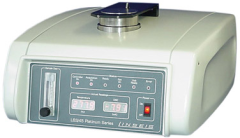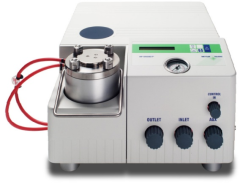# Calorimeters and Thermal Analyzers Information

Specific heat capacity is the measurement of heat a gram of a given substance needs to exchange with its surroundings under certain conditions in order to change its temperature by one degree. Calorimeters and thermal analyzers contain a heat source that can deliver a heat flux, at a distinct temperature, into a sample, and a temperature-measuring device that can read the resultant change in temperature. In order to obtain correct measurements, it is essential that the sample be prevented from exchanging any additional energy with its surroundings. When the heat source is in the off position, the temperature of the sample remains constant. When the heat source is activated for a given time interval, an exchange of energy in the form of heat takes place and the temperatureof the sample rises. The calorimeter or thermal analyzer calculates the specific heat capacity from the heat flux, the time, and the resulting change in the temperature of the sample.

## Types

There are a number of calorimeters and thermal analyzers available, which function using slightly different techniques.  The most common types of calorimeters and thermal analyzers include dilatometers, differential thermal analyzers (DTA), dynamic mechanical analyzers (DMA), thermal conductivity analyzers (TCA), thermal gravimetric analyzers (TGA), and thermal mechanical analyzers (TMA).

• Dilatometers are normally used to measure the expansion or shrinkage of solids with changing temperatures.
• Differential thermal analysis is the measurement of the difference in temperature between a sample and a reference as heat is applied to the system. This method is sensitive to endothermic and exothermic processes including: phase transitions, dehydration, and decomposition, redox, or solid-state reactions.
• Dynamic mechanical analysis (DMA) is an analytical technique, which measures the modulus (stiffness) and damping (energy dissipation) properties of materials as those materials are deformed under periodic (oscillatory) stress. There are several components, which are critical to the design and resultant performance of a dynamic mechanical analyzer. These components are the drive motor (which supplies the sinusoidal deformation force to the sample material), the drive shaft support and guidance system (which transfers the force from the drive motor to the clamps which hold the sample), the displacement sensor (which measures the sample deformation [oscillation amplitude] that occurs under the applied force), the temperature control system (furnace), and the sample clamps.
• The thermal conductivity is the quantity of heat transmitted, due to unit temperature gradient, in unit time under steady conditions in a direction normal to a surface of unit area, when the heat transfer is dependent only on the temperature gradient.
• TGA is the second most commonly used thermal technique. It measures weight changes in a material as a function of temperature (or time) under a controlled atmosphere. Its principal uses include measurement of a material's thermal stability and composition. TGA instruments are routinely used in all phases of research, quality control and production operations.
• TMA is a technique used for measuring the dimensional changes in materials as a function of temperature or as a function of time isothermally. Stress can be applied to the sample during the analysis.  The technique involves the use of a probe resting on a sample under a positive load. As the sample is heated, cooled or held isothermally, dimensional changes are translated into the linear displacement of the probe. Various probes are available for either penetration or expansion.